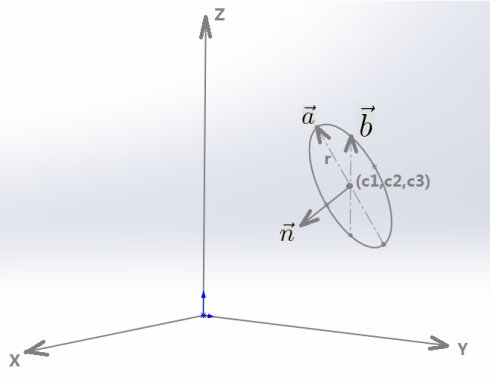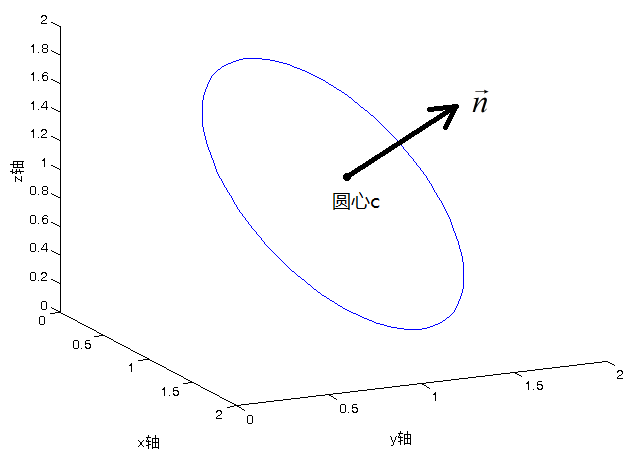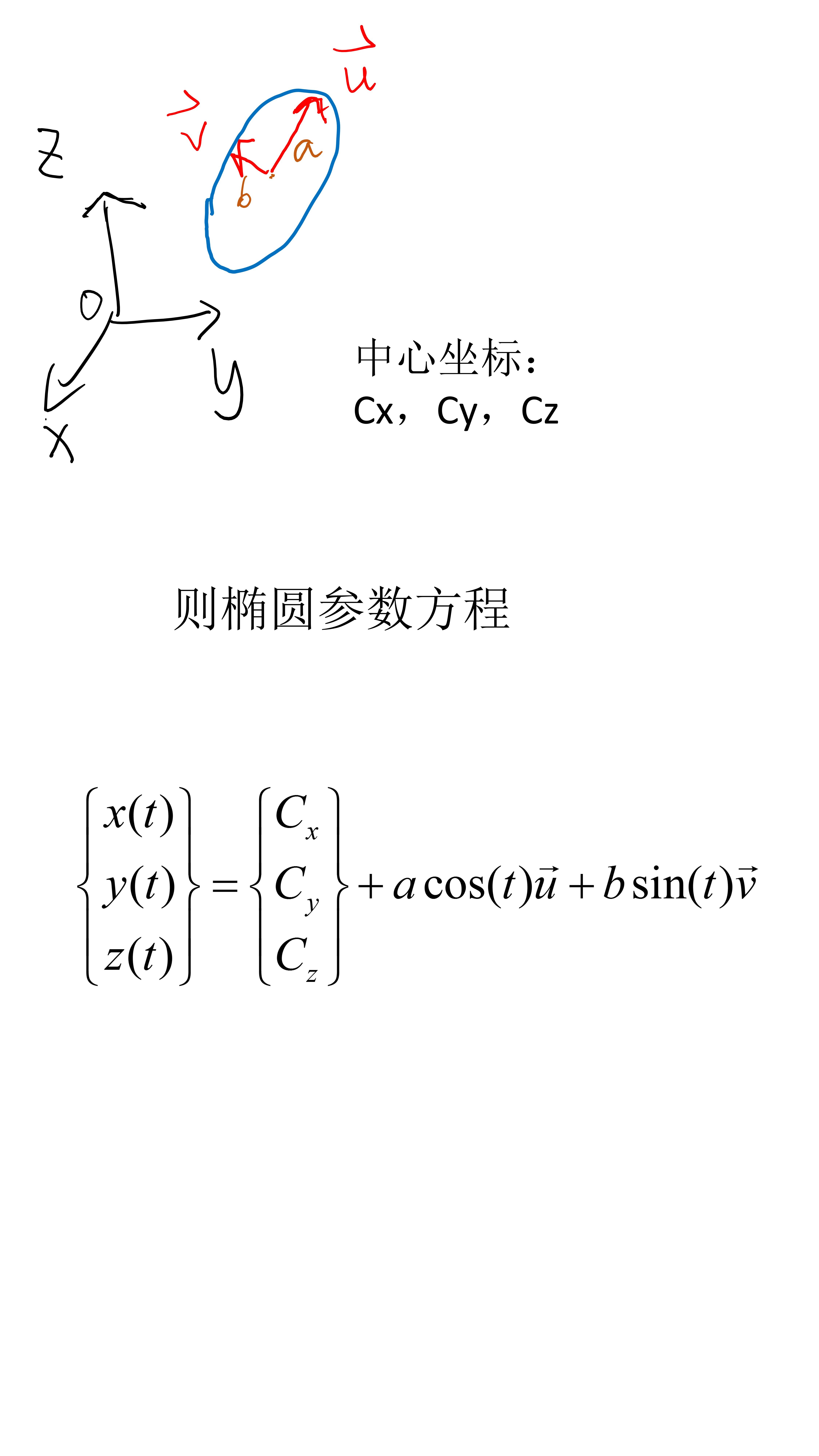• 原文链接： 三维空间中圆的参数方程 三维空间中，以点为圆心、以向量为法向量、半径为 r 的（见下图）， 它的参数方程为：其中，与分别对应单位向量与，它们既垂直于，又互相垂直；随着从0变化到，通过参数方程...
原文链接： 三维空间中圆的参数方程
三维空间中，以点为圆心、以向量为法向量、半径为 r 的圆（见下图），它的参数方程为：其中，与分别对应单位向量与，它们既垂直于，又互相垂直；随着从0变化到，通过参数方程可以得到圆上每一个点的坐标。与是满足既垂直于，又互相垂直的任意单位向量。怎么样快速得到满足条件的与呢？这时候应该充分利用叉乘运算的特点，因为两个向量的叉乘结果只要不为零，叉乘结果总是垂直于原来的这两个向量。具体如下：求的方法：叉乘坐标向量。如果叉乘结果不为零，那么它必然垂直于，把它单位化后就得到；如果叉乘结果恰好为零，再用叉乘剩下两个坐标向量与中任意一个，单位化叉乘结果，得到。求的方法：叉乘上一步得到的，叉乘结果必然垂直于与，单位化叉乘结果，就得到。接下来，利用Matlab软件对三维空间中圆的参数方程进行测试。代码如下：n=[1 1 1]; %法向量nr=1; %圆的半径为1c=[1 1 1]; %圆心的坐标theta=(0:2*pi/100:2*pi)'; %theta角从0到2*pia=cross(n,[1 0 0]); %n与i叉乘，求取a向量if ~any(a) %如果a为零向量，将n与j叉乘     a=cross(n,[0 1 0]);endb=cross(n,a); %求取b向量a=a/norm(a); %单位化a向量b=b/norm(b); %单位化b向量c1=c(1)*ones(size(theta,1),1);c2=c(2)*ones(size(theta,1),1);c3=c(3)*ones(size(theta,1),1);x=c1+r*a(1)*cos(theta)+r*b(1)*sin(theta);%圆上各点的x坐标y=c2+r*a(2)*cos(theta)+r*b(2)*sin(theta);%圆上各点的y坐标z=c3+r*a(3)*cos(theta)+r*b(3)*sin(theta);%圆上各点的z坐标plot3(x,y,z)xlabel('x轴')ylabel('y轴')zlabel('z轴')Matlab运行结果如下图：展开全文•展开全文• 三维空间中，以点为圆心、以向量为法向量、半径为 r 的（见下图）， 它的参数方程为：其中，与分别对应单位向量与，它们既垂直于，又互相垂直；随着从0变化到，通过参数方程可以得到上每一个点的坐标。与是...
三维空间中，以点为圆心、以向量为法向量、半径为 r 的圆（见下图），它的参数方程为：其中，与分别对应单位向量与，它们既垂直于，又互相垂直；随着从0变化到，通过参数方程可以得到圆上每一个点的坐标。与是满足既垂直于，又互相垂直的任意单位向量。怎么样快速得到满足条件的与呢？这时候应该充分利用叉乘运算的特点，因为两个向量的叉乘结果只要不为零，叉乘结果总是垂直于原来的这两个向量。具体如下：求的方法：叉乘坐标向量。如果叉乘结果不为零，那么它必然垂直于，把它单位化后就得到；如果叉乘结果恰好为零，再用叉乘剩下两个坐标向量与中任意一个，单位化叉乘结果，得到。求的方法：叉乘上一步得到的，叉乘结果必然垂直于与，单位化叉乘结果，就得到。接下来，利用Matlab软件对三维空间中圆的参数方程进行测试。代码如下：n=[1 1 1]; %法向量nr=1; %圆的半径为1c=[1 1 1]; %圆心的坐标theta=(0:2*pi/100:2*pi)'; %theta角从0到2*pia=cross(n,[1 0 0]); %n与i叉乘，求取a向量if ~any(a) %如果a为零向量，将n与j叉乘     a=cross(n,[0 1 0]);endb=cross(n,a); %求取b向量a=a/norm(a); %单位化a向量b=b/norm(b); %单位化b向量c1=c(1)*ones(size(theta,1),1);c2=c(2)*ones(size(theta,1),1);c3=c(3)*ones(size(theta,1),1);x=c1+r*a(1)*cos(theta)+r*b(1)*sin(theta);%圆上各点的x坐标y=c2+r*a(2)*cos(theta)+r*b(2)*sin(theta);%圆上各点的y坐标z=c3+r*a(3)*cos(theta)+r*b(3)*sin(theta);%圆上各点的z坐标plot3(x,y,z)xlabel('x轴')ylabel('y轴')zlabel('z轴')Matlab运行结果如下图：展开全文• 表达式 证明 特点 一般式 A1x+B1y+C1z+D1=0 A2x+B2y+C2z+D2=0 两个平面相交的交线。... 直线的方向是两个... A(x0,y0,z0)是空间的一点，向量s(m,n,p)为非零向量且与直线l平行，B(x,y,z...
 表达式证明特点一般式 A1x+B1y+C1z+D1=0 A2x+B2y+C2z+D2=0 两个平面相交的交线。直线的方向是两个平面法向量的外积。点向式（对称式）(x-x0)/m=(y-y0)/n=(z-z0)/pA(x0,y0,z0)是直线上的一点，向量s(m,n,p)为非零向量且与直线l平行，B(x,y,z)是直线上任意一点，向量AB与向量s平行，即向量的各个分量成比例，于是有(x-x0)/m=(y-y0)/n=(z-z0)/p 1、方向向量是(m,n,p)； 2、过点(x0,y0,z0)。 参数方程 x=x0+mt y=y0+nt z=z0+pt 有直线(x-x0)/m=(y-y0)/n=(z-z0)/p，令t=(x-x0)/m=(y-y0)/n=(z-z0)/p，则有： x=x0+mt y=y0+nt z=z0+pt  1、方向向量是(m,n,p)； 2、过点(x0,y0,z0)。
展开全文• ## 图解圆的参数方程

万次阅读 2018-06-15 17:11:02
• 本代码实现了对三维空间中任意不共线三点确定一个园的算法进行代码实现，并针对工程应用，详细的描述了其圆周的参数方程描述，对当前工业应用的三维空间中的圆周插补具有一个很好的帮助。
• 给出一种空间圆周的参数方程描述，便于计算机编程实现。
• Ax+By+Cz+D=0 (参数,A,B,C,D是描述平面空间特征的常数) 如何求参数： 选择逆时针凸多边形的个连续顶点(x1,y1,z1),(x2,y2,z2),(x3,y3,z3) 建立方程组来求A,B,C,D（为什么要选择凸多边形,凸多边形能保证，任意三点...
• 本文要解决如下问题：给定一些三维空间点，要求用直线拟合这些点，求出直线方程。 直线方程的三种表示方法： 1.一般式： 它实际上表示，直线是由经验分享
•向量空间 解析几何
• ## 椭圆3维空间方程式

千次阅读 2020-06-03 11:23:00
1、其中满足u垂直v垂直n(椭圆的法向量)，a为椭圆的长边半径，b为椭圆的短边半径 （Cx,Cy,Cz）是中心点坐标
•  参数方程（字母所代表含义可参见【第六回】OCCT之Package Geom的曲线曲面参数方程解析）： P(U) = O + R*Cos(U)*XDir + R*Sin(U)*YDir 这个问题主要应用于二多段线中圆弧段的求解。在这里我先讲一下二多...圆弧
•  参数方程（字母所代表含义可参见 【第六回】OCCT之Package Geom的曲线曲面参数方程解析 ）： P(U) = O + R*Cos(U)*XDir + R*Sin(U)*YDir  现在开始求解。  设圆弧上这三点分别为A,B,C，现在要求...内接三角形 向量空间 解析几何
• ## 空间三维直线方程求解方法

万次阅读 多人点赞 2018-01-17 10:22:48
2018-01-17 创建人：Ruo_Xiao 邮箱：xclsoftware@163.com 1、一般方程：两个相交的平面确定一条直线。 2、点向式：点和直线方向可以确定一条直线。 3、两点式：空间两个点确定一条直线。
• 参考链接：三维空间中的平面方程 这个链接是错误的： http://blog.csdn.net/PengPengBlog/article/details/52774421   //获取平面方程//Ax + By + Cz + D std::vector&lt;float&gt; getPlaneParam(const...
• 请问一下，如果已知个点的带参数坐标（坐标带有两个变量参数参数不用求，要带在方程结果里) ，如何可以通过matlab求过着个点的方程呢？求指教~matlab
• 针对四阶椭圆方程，构造一个十四参数非协调四面体元，并在三维空间中证明了该单元关于重调和方程模型收敛。
• 维空间下椭圆基本方程为  (1) 这个是我们大家都熟知的，但是，如果背景空间不是二维空间，而是N维欧式空间中的椭圆，其表达式应该是什么样式的？ 为了对这个问题论证比较严格，在下述过程中采用了微分几何...数学 微分几何
• 4.共面之后就可以求交点了，我们列出参数方程 pa=ta*p1+(1-ta)*p2; pb=tb*p1+(1-tb)*p2; pa=pb; 解方程得出t1或者t2,代入原参数方程就是交点 5. t1和t2如果都在0到1范围内，则两个线段相交 就酱 ...计算几何
• 三维欧几里得空间中，直线的关系为：相交、平行和异面直线三种情况。本文采用向量方程来定义直线，向量的叉乘、点乘来计算直线间距离。程序用C++实现。C++ 线性代数 计算几何
• 介绍了彩色三维激光扫描测量系统的工作原理，推导出空间三维坐标的光平面方程测量模型。提出了系统结构参数的优化设计方法，进行了优化实验，根据实验结果，确定了测量系统的最佳参数值。基于这些参数建造的测量系统...
• 通过输入任意三点空间坐标自动判断三点是否在一条直线上，若不在则求三点外接的圆心位置和半径，计算精度可调节。解决了“知三点求圆心半径”程序对于某些点无法计算的缺陷。
• 但是在网上寻找资料的时候大多是建立在二维空间的，在三维坐标系下的拟合较乱。因此在下面列举一些我所用到的直线拟合和拟合。 空间拟合 在二维空间中对的拟合较为简单，由初中的几何知识我们可以知道，确定...
• 实际上知道二维空间中的求法（使用坐标系进行求解的），要想从中推导到三维空间中的求法是不容易的。在进行几何问题求解时，除了使用 坐标系求解问题 外，使用 向量求解问题 也许更容易。向量空间是隐藏坐标系的，...向量空间 两直线相交
• 本文内容包括： - 三维空间中射线与平面的表示方法， - 三维空间中判断射线与平面是否相交。计算机视觉 多视图几何
• 本文旨在阐释一种算法，用于在三维空间中寻找某一线(ray)与某一三角形的交点。此算法是计算机图形学中的基础算法之一。 1.预设概念 为了阐释此算法，必须先引入一组预设概念，借以使用计算机语言来描述三维空间中...
• 说到这个博客的题目，可能觉得有点大，在测绘学领域中三维空间坐标的相似变换用得非常多。那么什么是三维坐标的相似变换呢？就是在两个三维直角坐标系中，坐标进行变换，两个坐标系之间变换需要七个参数，即三个平移...
• ## 三维空间距离公式

千次阅读 2020-05-29 11:11:54
求点P到直线的距离 任取直线上一点M，支线方向向量为n，则 求点P到平面的距离 设平面方程为Ax+By+Cz+D=0，点P坐标为(X0,Y0,Z0)，则
• % 随机生成一组（x,y,z),这些点的坐标离一个空间平面比较近 x0=1; L1=2; y0=1; L2=2; x=x0+rand(20,1)*L1; y=y0+rand(20,1)*L2; z=1+2*x+3*y; scatter3(x,y,z,'filled') hold on; planeData=[x,y,z]; % 协方差矩阵......